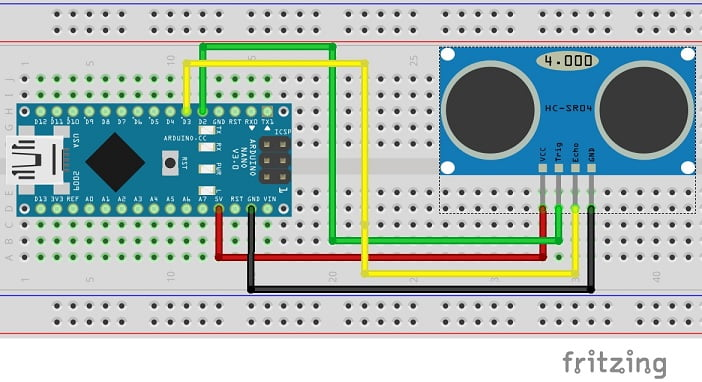# Ultrasonic Sensor

One of the easiest and more accurate ways of liquid measurement, the ultrasonic sensor. An ultrasonic sensor emits ultrasonic waves from the transmitter (emitter), these waves hit an object in the case of the agribot, its liquid measurement and then receives the reflected waves through the receiver (detector).Figure 1: How the ultrasonic sensor works, obstacle would be fluid level of tank.

#### Components Needed

All you will need for the Ultrasonic sensor measurement system will be:

• Breadboard
• Wires
• Ultrasonic Sensor
• Arduino

#### How to Wire The Ultrasonic Sensor to a Breadboard.

One of the main benefits of using the ultrasonic sensor is the fact that its very easy to wire into a circuit. The sensor has four pins. Left – Right, Vcc, Trig, Echo, GND.Figure 2: What the ultrasonic sensor looks like.

Vcc is the pin where you supply the voltage to the sensor. Trig pin is the trigger pin this receives the input form the arduino to send out a wave through the transmitter. The Echo pin is where we communicate back to the arduino through an electric signal got from the reflected wave. GND then is the ground pin.Figure 3: Same as the circuit we built but instead of D3, D2 we used D8 for echo and D9 for trig pins

#### The Code For The Ultrasonic Sensor.

`// Another HC-SR04 rangefinder example for Arduino Nano// Written by David Haran, Martin Olaseni, Mpoyi Tshivuila, Carmen Boupda, last updated 22/11/2020//// Arduino pins://// D8 is an input for the rangefinder's echo pin// D9 is an output for the rangefinder's trigger pin//`

`include`` <math.h>`

`double distance, volume_cube, volume_liquid_cube, volume_free_cube, volume_cylinder, volume_liquid_cylinder, volume_free_cylinder;`

`void setup()`
`{`
`pinMode(8, OUTPUT); // echo pinpinMode(9, OUTPUT); // trigger pin`

`// Open a serial connection to display the distance measurementsSerial.begin(9600);}`

`void loop(){// Declare variablesunsigned long tus; // time of flight in microsecondsdouble c = 34300.0; // speed of sound in centimetres per seconddouble d; // distance to object in metresdouble volume_cylinder;double volume_liquid_cylinder;double volume_free_cylinder;float r = 18;float h_cyl = 26;`

`// Set trigger pulsedigitalWrite(9, HIGH); // set TRIG to 5VdelayMicroseconds(200);digitalWrite(9, LOW); // set TRIG to 0V`

`// Use the pulseIn Arduino function to measure the duration// of the echo pulse in microsecondstus = pulseIn(8, HIGH, 50000);`

`// Calculate the distance in metresd = tus * (c / 2e6);`

`// Print out the distance over the serial connection// To view the printed text, open the "Tools" menu in// the Arduino IDE and select "Serial Monitor".//Serial.print("distance = ");//Serial.print(d);//Serial.println(" metres");`

`// Leave some time before loop function// runs again to allow any ultrasound// in the room to die away before next// measurement. Otherwise, successive// pulses will get mixed up!// delay(100);`

## `//If cube used for tank`

`//volume_cube = wlh; //calculate volume of cube//volume_free_cube = wld; //calculate volume of free space in the cube//volume_liquid_cube = volume_cube - volume_free_cube; //volume of cube - volume of free space = area of liquid in the tank//Serial.print(volume_liquid_cube); //print value for the volume of liquid//Serial.println(" cm^3"); //print unit`

## `//If tank is a cylinder`

`volume_cylinder = PI * pow(r, 2) * h_cyl; //calculate volume of cylindervolume_free_cylinder = PI * pow(r, 2) * d; //calculate volume of free space in the cylindervolume_liquid_cylinder = volume_cylinder - volume_free_cylinder; //volume - volume of free space = area of liquid in the tankSerial.print("Volume = ");Serial.print(volume_liquid_cylinder); //print value for the volume of liquidSerial.println(" cm^3"); //print unitdelay(100);}`

Depending on the shape of the tank that you use will depend on which piece of the code you use if you are using a cylindrical shaped take you don’t need to change the code apart from the two floats r and h_cyl, radius and height of the cylinder, yo match your fuel tank. And then use the code in blue for the cylinder.

If you are using a cube tank you will need to remove the floats r and h_cyl and add two l and w, for length and width of your fuel tank. You will then use the red code for the cube tank.

#### REFERENCES:

https://www.fierceelectronics.com/sensors/what-ultrasonic-sensor // Used to get to wave image.

https://www.botshop.co.za/wp-content/uploads/2016/09/HC-SR04-Ultrasonic-Sensor.jpg // Image of ultrasonic sensor.

https://www.officialhrm.com/arduino/arduino-hc-sr04 // For image of circuit

Create your website with WordPress.com